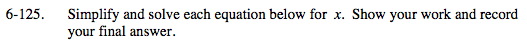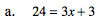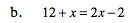### Home > MC2 > Chapter 6 > Lesson 6.2.6 > Problem6-125

6-125.Begin isolating the variable by subtracting 3 from each side.

24 = 3x + 3
−3 −3

21 = 3x

Divide each side by 3.

$x = \frac{21}{3} = 7$Follow the method of isolating the variable as presented in (a).